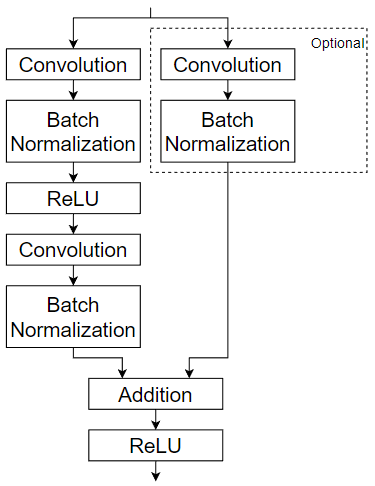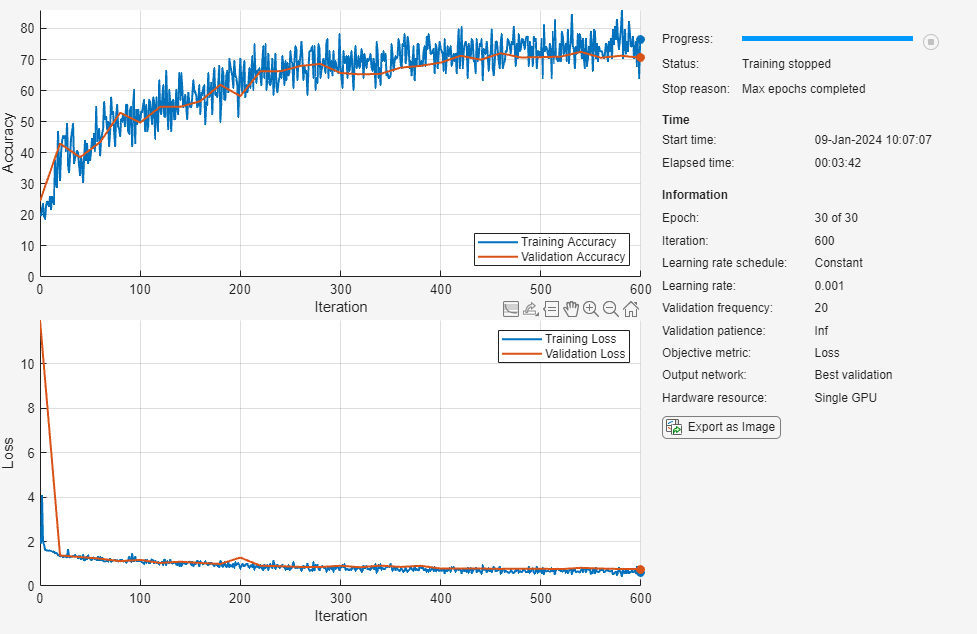# Train Deep Learning Network with Nested Layers

This example shows how to train a network with nested layers.

To create a custom layer that itself defines a layer graph, you can specify a `dlnetwork` object as a learnable parameter. This method is known as network composition. You can use network composition to:

• Create a single custom layer that represents a block of learnable layers, for example, a residual block.

• Create a network with control flow. For example, a network with a section that can dynamically change depending on the input data.

• Create a network with loops. For example, a network with sections that feed the output back into itself.

This example shows how to train a network using custom layers representing residual blocks, each containing multiple convolution, group normalization, and ReLU layers with a skip connection. For an example showing how to create a residual network without using custom layers, see Train Residual Network for Image Classification.

Residual connections are a popular element in convolutional neural network architectures. A residual network is a type of network that has residual (or shortcut) connections that bypass the main network layers. Using residual connections improves gradient flow through the network and enables the training of deeper networks. This increased network depth can yield higher accuracies on more difficult tasks.

This example uses the custom layer `residualBlockLayer`, which contains a learnable block of layers consisting of convolution, group normalization, ReLU, and addition layers, and also includes a skip connection and an optional convolution layer and group normalization layer in the skip connection. This diagram highlights the residual block structure.For an example showing how to create the custom layer `residualBlockLayer`, see Define Nested Deep Learning Layer.

### Prepare Data

```url = "http://download.tensorflow.org/example_images/flower_photos.tgz"; downloadFolder = tempdir; filename = fullfile(downloadFolder,"flower_dataset.tgz"); imageFolder = fullfile(downloadFolder,"flower_photos"); if ~datasetExists(imageFolder) disp("Downloading Flowers data set (218 MB)...") websave(filename,url); untar(filename,downloadFolder) end```

Create an image datastore containing the photos.

```datasetFolder = fullfile(imageFolder); imds = imageDatastore(datasetFolder, ... IncludeSubfolders=true, ... LabelSource="foldernames");```

Partition the data into training and validation data sets. Use 70% of the images for training and 30% for validation.

`[imdsTrain,imdsValidation] = splitEachLabel(imds,0.7,"randomized");`

View the number of classes of the data set.

```classes = categories(imds.Labels); numClasses = numel(classes)```
```numClasses = 5 ```

Data augmentation helps prevent the network from overfitting and memorizing the exact details of the training images. Resize and augment the images for training using an `imageDataAugmenter` object:

• Randomly reflect the images in the vertical axis.

• Randomly translate the images up to 30 pixels vertically and horizontally.

• Randomly rotate the images up to 45 degrees clockwise and counterclockwise.

• Randomly scale the images up to 10% vertically and horizontally.

```pixelRange = [-30 30]; scaleRange = [0.9 1.1]; imageAugmenter = imageDataAugmenter( ... RandXReflection=true, ... RandXTranslation=pixelRange, ... RandYTranslation=pixelRange, ... RandRotation=[-45 45], ... RandXScale=scaleRange, ... RandYScale=scaleRange);```

Create an augmented image datastore containing the training data using the image data augmenter. To automatically resize the images to the network input size, specify the height and width of the input size of the network. This example uses a network with input size `[224 224 3]`.

```inputSize = [224 224 3]; augimdsTrain = augmentedImageDatastore(inputSize(1:2),imdsTrain,DataAugmentation=imageAugmenter);```

To automatically resize the validation images without performing further data augmentation, use an augmented image datastore without specifying any additional preprocessing operations.

`augimdsValidation = augmentedImageDatastore([224 224],imdsValidation);`

### Define Network Architecture

Define a residual network with six residual blocks using the custom layer `residualBlockLayer`. To access this layer, open the example as a live script. For an example showing how to create this custom layer, see Define Nested Deep Learning Layer.

Because you must specify the input size of the input layer of the `dlnetwork` object, you must specify the input size when creating the layer. To help determine the input size to the layer, you can use the `analyzeNetwork` function and check the size of the activations of the previous layer.

```numFilters = 32; layers = [ imageInputLayer(inputSize) convolution2dLayer(7,numFilters,Stride=2,Padding="same") groupNormalizationLayer("all-channels") reluLayer maxPooling2dLayer(3,Stride=2) residualBlockLayer(numFilters) residualBlockLayer(numFilters) residualBlockLayer(2*numFilters,Stride=2,IncludeSkipConvolution=true) residualBlockLayer(2*numFilters) residualBlockLayer(4*numFilters,Stride=2,IncludeSkipConvolution=true) residualBlockLayer(4*numFilters) globalAveragePooling2dLayer fullyConnectedLayer(numClasses) softmaxLayer classificationLayer]```
```layers = 15×1 Layer array with layers: 1 '' Image Input 224×224×3 images with 'zerocenter' normalization 2 '' 2-D Convolution 32 7×7 convolutions with stride [2 2] and padding 'same' 3 '' Group Normalization Group normalization 4 '' ReLU ReLU 5 '' 2-D Max Pooling 3×3 max pooling with stride [2 2] and padding [0 0 0 0] 6 '' Residual Block Residual block with 32 filters, stride 1 7 '' Residual Block Residual block with 32 filters, stride 1 8 '' Residual Block Residual block with 64 filters, stride 2, and skip convolution 9 '' Residual Block Residual block with 64 filters, stride 1 10 '' Residual Block Residual block with 128 filters, stride 2, and skip convolution 11 '' Residual Block Residual block with 128 filters, stride 1 12 '' 2-D Global Average Pooling 2-D global average pooling 13 '' Fully Connected 5 fully connected layer 14 '' Softmax softmax 15 '' Classification Output crossentropyex ```

### Train Network

Specify training options:

• Train the network with a mini-batch size of 128.

• Shuffle the data every epoch.

• Validate the network once per epoch using the validation data.

• Output the network with lowest validation loss.

• Display the training progress in a plot and disable the verbose output.

```miniBatchSize = 128; numIterationsPerEpoch = floor(augimdsTrain.NumObservations/miniBatchSize); options = trainingOptions("adam", ... MiniBatchSize=miniBatchSize, ... Shuffle="every-epoch", ... ValidationData=augimdsValidation, ... ValidationFrequency=numIterationsPerEpoch, ... OutputNetwork="best-validation-loss", ... Plots="training-progress", ... Verbose=false);```

Train the network using the `trainNetwork` function. By default, `trainNetwork` uses a GPU if one is available, otherwise, it uses a CPU. Training on a GPU requires Parallel Computing Toolbox™ and a supported GPU device. For information on supported devices, see GPU Computing Requirements (Parallel Computing Toolbox). You can also specify the execution environment by using the `ExecutionEnvironment` option of `trainingOptions`.

`net = trainNetwork(augimdsTrain,layers,options);`### Evaluate Trained Network

Calculate the final accuracy of the network on the training set (without data augmentation) and validation set. The accuracy is the proportion of images that the network classifies correctly.

```YPred = classify(net,augimdsValidation); YValidation = imdsValidation.Labels; accuracy = mean(YPred == YValidation)```
```accuracy = 0.7530 ```

Visualize the classification accuracy in a confusion matrix. Display the precision and recall for each class by using column and row summaries.

```figure confusionchart(YValidation,YPred, ... RowSummary="row-normalized", ... ColumnSummary="column-normalized");```You can display four sample validation images with predicted labels and the predicted probabilities of the images having those labels using the following code.

```idx = randperm(numel(imdsValidation.Files),4); figure for i = 1:4 subplot(2,2,i) I = readimage(imdsValidation,idx(i)); imshow(I) label = YPred(idx(i)); title("Predicted class: " + string(label)); end ```